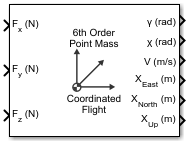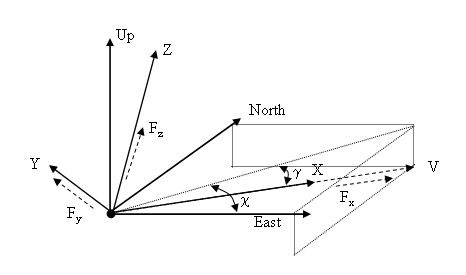# 6th Order Point Mass (Coordinated Flight)

Calculate sixth-order point mass in coordinated flight

• Library:
• Aerospace Blockset / Equations of Motion / Point Mass

•## Description

The 6th Order Point Mass (Coordinated Flight) block performs the calculations for the translational motion of a single point mass or multiple point masses. For more information on the system for the translational motion of a single point mass or multiple mass, see Algorithms.

The 6th Order Point Mass (Coordinated Flight) block port labels change based on the input and output units selected from the Units list.

## Limitations

• The block assumes that there is fully coordinated flight, i.e., there is no side force (wind axes) and sideslip is always zero.

• The flat Earth reference frame is considered inertial, an approximation that allows the forces due to the Earth motion relative to the "fixed stars" to be neglected.

## Ports

### Input

expand all

Force in x-axis, specified as a scalar or vector, in selected units.

Data Types: `double`

Force in y-axis, specified as a scalar or vector, in selected units.

Data Types: `double`

Force in z-axis, specified as a scalar or vector, in selected units.

Data Types: `double`

### Output

expand all

Flight path angle, returned as a scalar or vector, in radians.

Data Types: `double`

Data Types: `double`

Airspeed, returned as a scalar or vector, in selected units.

Data Types: `double`

Downrange or amount traveled east, returned as a scalar or vector, in selected units.

Data Types: `double`

Crossrange or amount traveled north, returned as a scalar or vector, in selected units.

Data Types: `double`

Altitude or amount traveled up, returned as a scalar or vector, in selected units.

Data Types: `double`

## Parameters

expand all

Input and output units, specified as:

Units

Forces

Velocity

Position

Mass

`Metric (MKS)`

newtons

meters per second

meters

kilograms

```English (Velocity in ft/s)```

pounds

feet per second

feet

slugs

```English (Velocity in kts)```

pounds

knots

feet

slugs

#### Programmatic Use

 Block Parameter: `units` Type: character vector Values: ```'Metric (MKS)'``` | ```'English (Velocity in ft/s)'``` | ```'English (Velocity in kts)'``` Default: ```'Metric (MKS)'```

Initial flight path angle of the point mass(es), specified as a scalar or vector.

#### Programmatic Use

 Block Parameter: `gamma0` Type: character vector Values: scalar | vector Default: `'0'`

Initial heading angle of the point mass(es), specified as a scalar or vector.

#### Programmatic Use

 Block Parameter: `chi0` Type: character vector Values: scalar | vector Default: `'0'`

Initial airspeed of the point mass(es), specified as a scalar or vector.

#### Programmatic Use

 Block Parameter: `V0` Type: character vector Values: scalar | vector Default: `'100'`

Initial downrange of the point mass(es), specified as a scalar or vector.

#### Programmatic Use

 Block Parameter: `x0` Type: character vector Values: scalar | vector Default: `'0'`

Initial crossrange of the point mass(es), specified as a scalar or vector.

#### Programmatic Use

 Block Parameter: `y0` Type: character vector Values: scalar | vector Default: `'0'`

Initial altitude of the point mass(es), specified as a scalar or vector.

#### Programmatic Use

 Block Parameter: `h0` Type: character vector Values: scalar | vector Default: `'0'`

Mass of the point mass(es), specified as a scalar or vector.

#### Programmatic Use

 Block Parameter: `mass0` Type: character vector Values: scalar | vector Default: `'1.0'`

## Algorithms

This figure shows the system for the translational motion of a single point mass or multiple point masses.The translational motion of the point mass [XEastXNorthXUp]T are functions of airspeed (V), flight path angle (γ), and heading angle (χ),

`$\begin{array}{l}{F}_{x}=m\stackrel{˙}{V}\\ {F}_{y}=\left(mV\mathrm{cos}\gamma \right)\stackrel{˙}{\chi }\\ {F}_{z}=mV\stackrel{˙}{\gamma }\\ {\stackrel{˙}{X}}_{East}=V\mathrm{cos}\chi \mathrm{cos}\gamma \\ {\stackrel{˙}{X}}_{North}=V\mathrm{sin}\chi \mathrm{cos}\gamma \\ {\stackrel{˙}{X}}_{Up}=V\mathrm{sin}\gamma \end{array}$`

where the applied forces [FxFyFh]T are in a system is defined by x-axis in the direction of vehicle velocity relative to air, z-axis is upward, and y-axis completes the right-handed frame, and the mass of the body m is assumed constant.

## Extended Capabilities

### C/C++ Code GenerationGenerate C and C++ code using Simulink® Coder™.

Introduced before R2006a

## SupportGet trial now